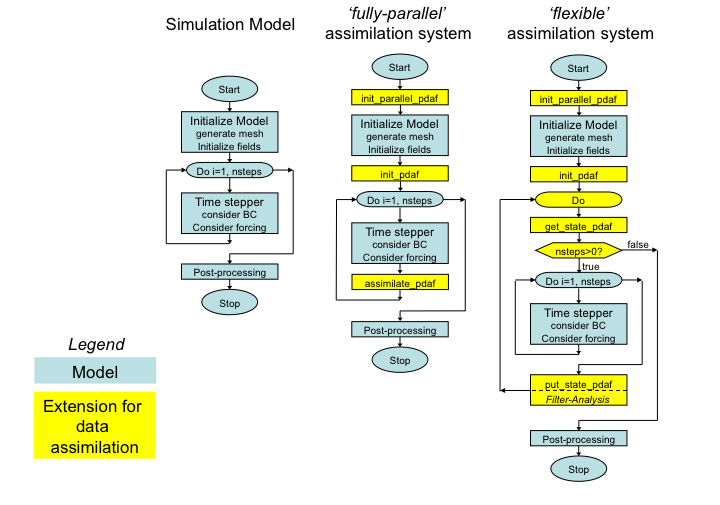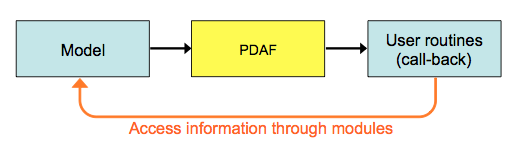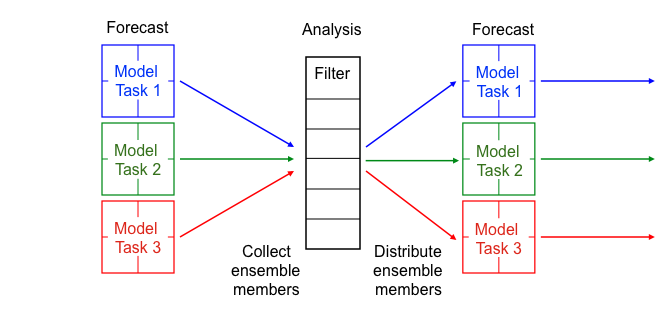# Implementation Concept of the Online Mode

#### Implementation Concept

1. General Concept
2. Online Mode
3. Offline Mode

## Online mode: Attaching PDAF to a model

Here we describe the extensions of the model code for the online mode of PDAF. The online mode offers two implementation variants. The first one, called fully-parallel, assumes that you have a sufficient number of processes when running the data assimilation program so that all ensemble states can be propagated concurrently. The parallelism allows for a simplified implementation. The second implementation variant, called flexible, allows to run the assimilation program in a way so that a model task (set of processors running one model integration) can propagate several ensemble states successively. This implementation variant is a bit more complicated, because one has to ensure that the model can jump back in time.

If the data assimilation can be run with a sufficient number of processors to use the 'fully-parallel' variant, we recommend to use it. With PDAF Version 1.10 particular interface routines `PDAF_assimilate_X` have been introduced to support this implementation style.

The assimilation system is built by adding calls to PDAF-routines to the general part of the model code. As only minimal changes to the model code are required, we refer to this as "attaching" PDAF to the model.

The general concept is depicted in figure 1. The left hand side shows a typical abstract structure of a numerical model. When the program is executed, the following steps are performed:

1. The model is initialized. Thus arrays for the model fields are allocated and filled with initial fields. Thus, the model grid is build up virtually in the program.
2. After the initialization the time stepping loop is performed. Here the model fields are propagated through time.
3. When the integration of the model fields is completed after a defined number of time steps, various post-processing operations are performed. Then the program stops.Figure 1: (left) Generic structure of a model code, (center) extension for fully-parallel data assimilation system with PDAF, (right) extension for flexible data assimilation system with PDAF.

Extensions for the fully-parallel assimilation system
The center of Figure 1 shows the extensions required for the fully-parallel assimilation system (marked yellow):

• `init_parallel_pdaf`: This routine is inserted close to the start of the model code. If the model itself is parallelized the correct location is directly after the initialization of the parallelization in the model code. `init_parallel_pdaf` creates the parallel environment that allows to perform several time stepping loops ("model tasks") at the same time.
• `init_pdaf`: This routine is added after the initialization part of the model. In `init_pdaf`, parameters for PDAF can be defined and then the core initialization routine `PDAF_init` is called. This core routine also initializes the array of ensemble states. Subsequently, the PDAF-core routines `PDAF_get_state` is called. This routine initializes model fields from the array of ensemble states and initializes the number of time steps that have to be computed and ensures that the ensemble integration is performed correctly.
• `assimilate_pdaf`: This routine is added to the model code at the end of the time stepping loop (just before the END DO in a Fortran program). The routine declares the names of user-supplied routines and calls a filter-specific PDAF-core routine `PDAF_assimilate_X` (with, e.g., X=estkf, for the ESTKF). This routine has to be called at the end of each time step. It counts whether all time steps of the current forecast phase have been computed. IF this is not the case, the program continues integrating the model. IF the forecast phase is completed, the analysis step (i.e. the actual assimilation of the observations) is computed. Subsequently, the next forecast phase is initialized by writing the analysis state vector into the model fields and setting the number of time steps in the next forecast phase. (Please note: The routines `PDAF_assimilate_X` have been introduced with Version 1.10 of PDAF.)

Extensions for the flexible assimilation system
The right hand side of Figure 1 shows the extensions required for the flexible assimilation system (marked yellow):

• `init_parallel_pdaf`: This routine is inserted close to the start of the model code. If the model itself is parallelized the correct location is directly after the initialization of the parallelization in the model code. `init_parallel_pdaf` creates the parallel environment that allows to perform several time stepping loops ("model tasks") at the same time.
• `init_pdaf`: This routine is added after the initialization part of the model. In `init_pdaf`, parameters for PDAF can be defined and then the core initialization routine `PDAF_init` is called. This core routine also initializes the array of ensemble states.
• Ensemble loop: In order to allow for the integration of the state ensemble an unconditional loop is added around the time stepping loop of the model. This loop will allow to compute the time stepping loop multiple time during the model integration. PDAF provides an exit-flag for this loop. (This external loop can be avoided with the fully-parallel implementation variant.)
• `get_state_pdaf`: Inside the ensemble loop, a call to the interface routine is added to the code. In this routine the names of user-supplied routines are declared and the PDAF-core routine `PDAF_get_state` is called. This routine initializes model fields from the array of ensemble states and initializes the number of time steps that have to be computed and ensures that the ensemble integration is performed correctly.
• `put_state_pdaf`: At the end of the external loop, the call to the interface routine `put_state_pdaf` is added to the model code. The routine declares the names of user-supplied routines and calls a PDAF_core routine that is specific for each filter. E.g. for the ESTKF, the routine `PDAF_put_state_estkf` is called. This routine writes the propagated model fields back into a state vector of the ensemble array. Also it checks whether the ensemble integration is complete. If not, the next ensemble member will be integrated. If the ensemble integration is complete, the analysis step (i.e. the actual assimilation of the observations) is computed.

With the implementation strategy of PDAF, calls to three to four routines have to be added to the model code. In case of the flexible implementation variant also the external loop has to be added. While this looks like a large change in figure 1, this change does actually only affect the general part of the model code. In addition, the amount of source code of the numerical model will be much longer than the addition for the data assimilation system. Please note that the calls to the routines `PDAF_get_state`, `PDAF_put_state_X`, `PDAF_assimilate_X` (X chosen according to the filter) could also be added directly in the model code. However, using the interface routines (`get_state_pdaf` and `put_state_pdaf`) reduces the additions to the model code.

## Important aspects of the implementation concept

• The implementation concept of PDAF does not require that the time stepping part of the model is implemented as a subroutine. Instead calls to subroutines that control of the ensemble integration are added to the model code before and after the code parts performing the time stepping. If the time stepping part is implemented as a subroutine, this subroutine can be called in between the additional routines.
• Depending on the parallelization, there can be cases in which the model has to jump back in time and cases in which the time always moves forward:
• Jumping back in time will be required if the number of model tasks used to evolve the ensemble states is smaller than the number of ensemble members. In this case a model task has integrate more than one model state and will have to jump back in time after the integration of each ensemble member. This case requires to implement the flexible implementation variant.
• If there are as many model tasks as ensemble members, the model time always moves forward. In this case, one can implement PDAF with the fully-parallel variant without the external ensemble loop. That is, one calls `PDAF_assimilate_X` at the end of each time step. This strategy might also be simpler if a model uses nested loops (like a loop over minutes inside a loop over hours).
• Model-specific operations like the initialization of the array of ensemble states in `PDAF_init` are actually performed by user-supplied routines. These routines are called through the standard interface of PDAF. Details on the interface and the required routines are given on the pages describing the implementation steps.
• The assimilation system is controlled by the user-supplied routines that are called through PDAF as call-back routines. With this strategy, the assimilation program is essentially driven by the model part of the program. Thus, logically the model is not a sub-component of the assimilation system, but the implementation with PDAF results in a model extended for data assimilation.Figure 2: Use of user-supplied call-back functions in PDAF

• The user-supplied call-back routines can be implemented in the context of the model analogously to the model code. For example, if the model is implemented using Fortran common blocks or modules of the model code, these can be used to implement the user-supplied routines, too. This simplifies the implementation of the user-supplied routines knowing about the particularities of their model.

## Parallelization of the data assimilation program

PDAF adds the possibility to perform parallel ensemble forecasts, even for models that by themselves do not use parallelization. The structure of the parallelized data assimilation program is displayed in figure 2. In the forecast phase of the data assimilation application, several model state integrations can be performed at the same time by several model tasks. If the numerical model it parallelized by itself, the parallel ensemble forecast adds a second level of parallelization. For the analysis step, in which the filter combines the ensemble of model states with the observations, PDAF provides several parallelized filter algorithms. If the model uses domain decomposition for the parallelization, the same decomposition is typically used in the filter. Before the analysis step, all ensemble members are gathered by the processes that compute the filter analysis. Subsequently to the analysis step, the ensemble members are distributed to all model tasks to enable the next parallel ensemble forecast. These operations are performed within PDAF, so that a user can directly benefit from the second level of parallelization. For the required extension of the parallelization configuration of the model a fully implemented template routine is provided with PDAF. The adaptation of the parallelization is described in the Implementation Guide.Figure 3: Two-level parallelization of PDAF: During the forecast phase several model tasks can be concurrently performed, while each model can be parallelized by itself. In the analysis step one the parallelized filter included in PDAF is applied.